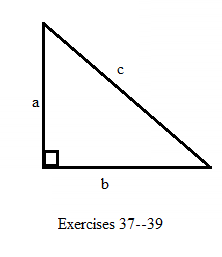Chapter A.5, Problem 38EElementary Geometry For College St...

7th Edition
Alexander + 2 others
ISBN: 9781337614085

Solutions

Chapter
SectionElementary Geometry For College St...

7th Edition
Alexander + 2 others
ISBN: 9781337614085
Textbook Problem

In Exercises 37-39, c 2 = a 2 + b 2 .In the right triangle, find b if a = 6     a n d     c = 10 .To determine

To find:

To find b if a=6andc=10.

Explanation

Consider the following equation,

c2=a2+b2.

The diagrammetic representation is given below,

Substitute the values of a=6andc=10 in the above equation to get the following,

c2=a2+b2102=62+b2100=36+

Still sussing out bartleby?

Check out a sample textbook solution.

See a sample solution

The Solution to Your Study Problems

Bartleby provides explanations to thousands of textbook problems written by our experts, many with advanced degrees!

Get Started

In Problem 1-10, find the real solutions to each quadratic equation.

Mathematical Applications for the Management, Life, and Social Sciences

Explain why the formula for sample variance is different from the formula for population variance.

Essentials of Statistics for The Behavioral Sciences (MindTap Course List)

Rewrite 10x2xdx using a trigonometric substitution. a) cos10sind b) 110sincos2d c) 10cos2sind d) 10sincosd

Study Guide for Stewart's Single Variable Calculus: Early Transcendentals, 8th